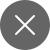# NumPy Reshape: 9 most important techniques for every Python beginner-Part 2

7+

Hi all, I hope you all are safe and sound in these times of distress and are recreating, reskilling yourself in this worldwide lockdown because of the pandemic. In the previous part(click here for Part 1), I had discussed some basic NumPy techniques that can be used if you are starting out as a Python programmer.

I mentioned matrix multiplication, transpose, how to calculate mean, median, standard deviation, etc. In this, I’ll be talking about more basic operations involved in NumPy like shape, reshape arrays in 3D, arrange function, zeros, etc.

Reshaping is one of the most important techniques that is used in machine learning and deep learning. Later on, when we’ll learn machine learning and deep learning we will understand how extensively matrices are used, especially in deep learning’ neural networks.

Let’s start

There are a lot of other ways to create arrays using NumPy. For eg using np.arrange().

One of the most important parts of machine learning is the reshaping of the matrix. Suppose we have a 2×5 matrix we can reshape it to 5×2.

We can create an identity matrix and we can create a zero and one matrix of any matrix dimension.

Now let’s move on to a bit more complex algorithm using np.linspace(). In this function, the function returns evenly spaced numbers at a given interval.

Other matrices operations that were not mentioned in Part 1 of the article.

We can also use NumPy to generate n number of random numbers and also access using basic slicing and dicing technique.

To generate random numbers in a 5×5 matrix and in 10×5 matrix.

How to access entries, multiple entries in a NumPy random matrix.

Accessing multiple non-consecutive entries using np.arrange().

For link to previous article click here. This ends our tutorial of NumPy basics using Python. Now, you can download a basic data set from any source and play with data using these NumPy codes. All the operations have been performed using Jupyter Notebook.7+

### 2 Responses

1. October 15, 2020

[…] NumPy […]

0
2. December 21, 2020

[…] NumPy Reshape: 9 most important techniques for every Python beginner-Part 2 – datamahadev.com. Hi all, I hope you all are safe and sound in these times of distress and are recreating, reskilling yourself in this worldwide lockdown because of the pandemic. […]

0